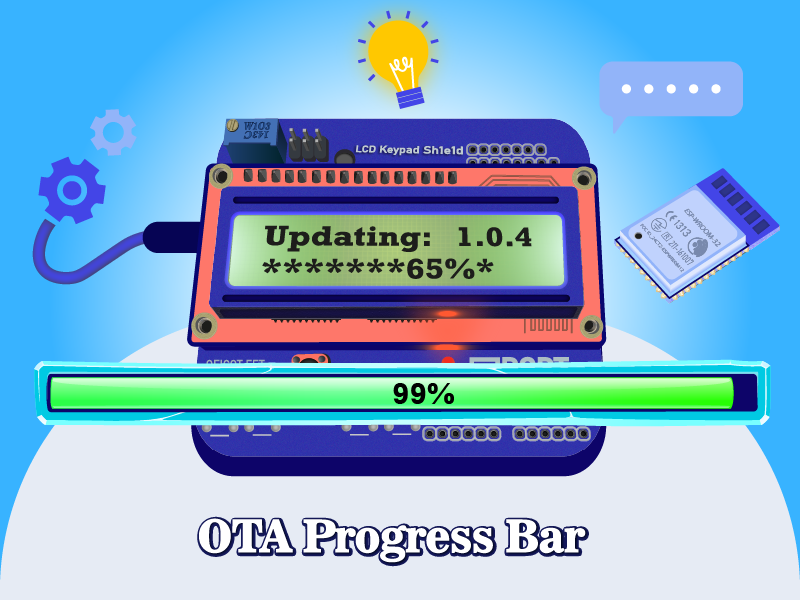## Introduction

It is popular to show a progress bar to the user while a lengthy operation is in progress. Formare OTA upgrade is one of the lengthy operations. It may take from 5 seconds up to one minute to complete. It can make the user bored and the user concludes that the device is stopped working and do a hard reset.

OTAdrive Arduino library provides a progressbar mechanism to the developers in order to notify the user the operation is going on and how much it has been done.
You should provide a callback handler method to the library to get the progress changes.

``````#include <otadrive_esp.h>

void OnProgress(int progress, int total);

void setup()
{
// Register your firmware info to the library
// Register your update progrees handler
...
}

void loop()
{
if (WiFi.status() == WL_CONNECTED && OTADRIVE.timeTick(10))
{
if (inf.available)
{
lcd.printf("Updating: %s", inf.version.c_str());
}
}
}

void OnProgress(int progress, int total)
{
// Show something to the user via LED or LCD
static int last_percent = 0;
int percent = (100 * progress) / total;
if (percent != last_percent)
{
for (uint8_t i = 0; i < percent; i+=6)
lcd.print("*");
}
}
``````

## Tutorial Video

The following video shows how to show the use an upadate progress bar while downloading OTA firmware in ESP32 or ESP8266. The code is same in both ESP32 and ESP8266 modules.

## Source Code

You can find the full project and source code of this project Here or see the main.c file following:

``````#include <LiquidCrystal.h>

// LCD pin to ESP32
const int pin_RS = 12;
const int pin_EN = 13;
const int pin_d4 = 17;
const int pin_d5 = 16;
const int pin_d6 = 27;
const int pin_d7 = 14;
const int pin_BL = -1;

LiquidCrystal lcd(pin_RS, pin_EN, pin_d4, pin_d5, pin_d6, pin_d7);
void OnProgress(int progress, int total);

void setup()
{

lcd.begin(16, 2);
lcd.setCursor(0, 0);
lcd.setCursor(0, 1);
lcd.print("App. V1.0.5");
delay(1000);
lcd.clear();
lcd.setCursor(0, 0);
lcd.print("App. V1.0.5");

}

int dummyCounter = 0;

void loop()
{
lcd.setCursor(0, 1);
lcd.printf("CNT %d", dummyCounter);
dummyCounter++;

if (WiFi.status() == WL_CONNECTED)
{
lcd.setCursor(7, 1);
lcd.print("WIFI");

{
if (inf.available)
{
lcd.clear();
lcd.setCursor(0, 0);
lcd.printf("Updating: %s", inf.version.c_str());
}
}
}
delay(500);
}

void OnProgress(int progress, int total)
{
static int last_percent = 0;
int percent = (100 * progress) / total;
if (percent != last_percent)
{
lcd.setCursor(0, 1);
for (uint8_t i = 0; i < percent; i+=6)
lcd.print("*");
lcd.setCursor(7, 1);
lcd.printf("%d%%", percent);
last_percent = percent;
}
}
``````